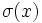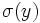# Preserves conjugacy classes for a generating set not implies class-preserving

This article gives the statement and possibly, proof, of a non-implication relation between two automorphism properties. That is, it states that every automorphism satisfying the first automorphism property (i.e., automorphism that preserves conjugacy classes for a generating set) need not satisfy the second automorphism property (i.e., class-preserving automorphism)
View a complete list of automorphism property non-implications | View a complete list of automorphism property implications
Get more facts about automorphism that preserves conjugacy classes for a generating set|Get more facts about class-preserving automorphism

## Statement

It is possible to have a group$G$, a generating set$S$ for$G$, and an automorphism$\sigma$ of$G$ such that$\sigma(x)$ is in the conjugacy class of$x$ (in$G$) for all$x \in S$, but there exists$y \in G, y \notin S$ such that$\sigma(y)$ is not conjugate to$y$.

## Proof

### Finite group examples

#### Alternating group of degree five

Further information: alternating group:A5, element structure of alternating group:A5

We can take$G$ to be alternating group:A5, the alternating group of degree five. For convenience, we take this to be the alternating group on the set$\{ 1,2,3,4,5 \}$.

We take$S$ to be the conjugacy class of$(1,2)(3,4)$ in$G$. This set has size 15 and is precisely the set of elements of$G$ that have order exactly two. Therefore, it is preserved under any automorphism. Moreover, it is easy to see that this set generates$G$ (in fact,$G$ has no proper subgroups of order equal to or larger than the size of this subset).

We take$\sigma$ to be any outer automorphism of$G$, such as that induced by any odd permutation in$S_5$. For instance, we can take$\sigma$ to be the outer automorphism induced by conjugation by$(1,2)$.

Now:

• As noted above,$S$ is a generating set of$G$ and is invariant under all automorphisms. It is also a single conjugacy class. Thus,$\sigma(x)$ is conjugate to$x$ for all$x \in S$.
• If we take$y = (1,2,3,4,5)$, then$\sigma(y)$ is not conjugate to$y$. This is because the conjugacy classes in the symmetric group of even permutations that correspond to partitions with distinct odd parts split inside the alternating group (see splitting criterion for conjugacy classes in the alternating group).

#### More general examples

Finite simple non-abelian groups are good sources of examples. In general, the following strategy works for a finite simple non-abelian group$G$ :

• Find a conjugacy class$S$ that is preserved under all automorphisms. Since the group is simple, this conjugacy class must generate the whole group.
• Find another conjugacy class that is not preserved under all automorphisms, and pick an outer automorphism$\sigma$ that does not preserve it.

For instance, the alternating group$A_n, n \ge 5$ always gives such examples. For$n \ne 6$, this follows immediately from the fact that the automorphism group is$S_n$, combined with the splitting criterion for conjugacy classes in the alternating group. The statement also holds for alternating group:A6 ($n = 6$), though it needs more careful examination of the element structure of alternating group:A6.